# Large Printable Number Line To 20Updated on Jun 28, 2022By Printablee Team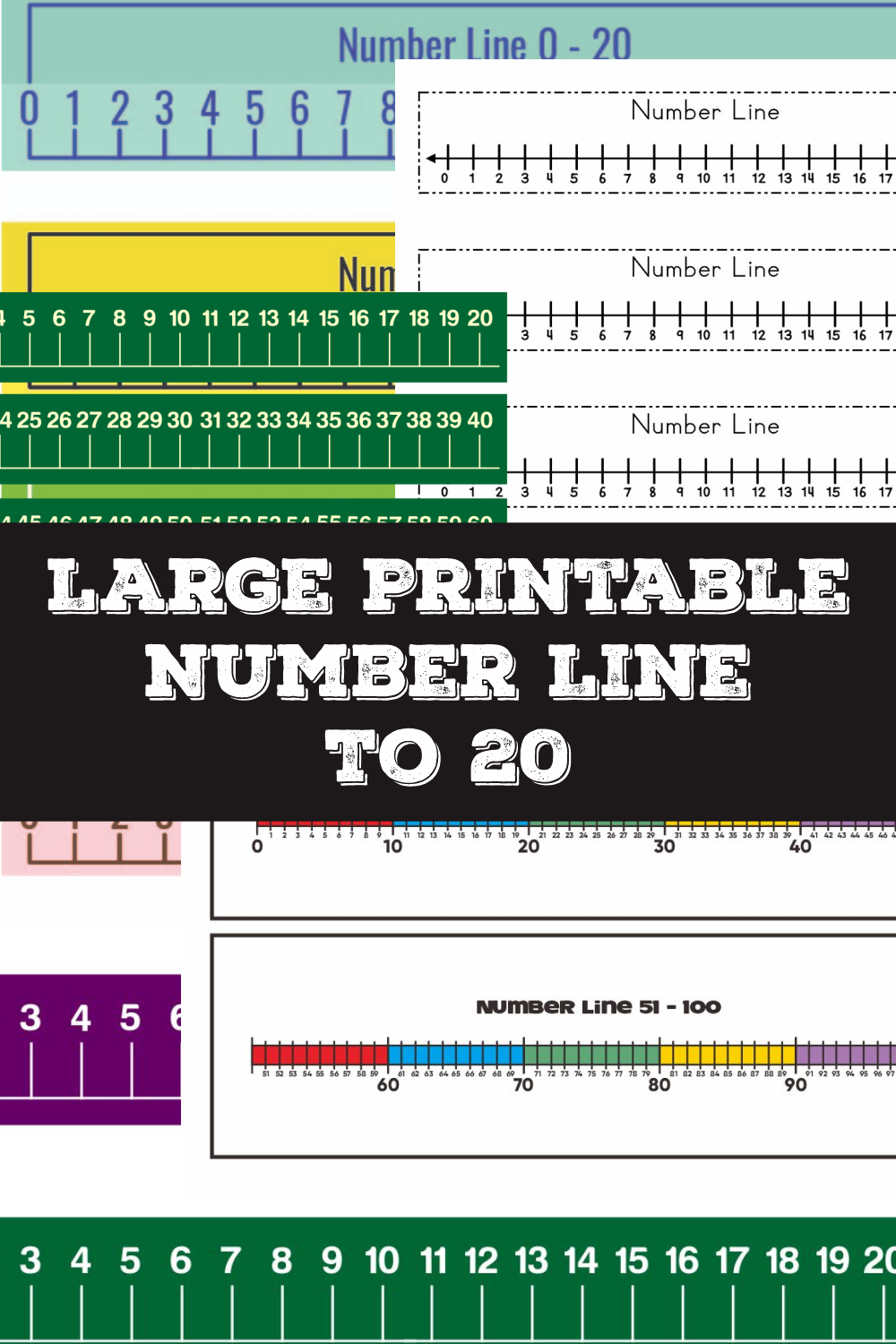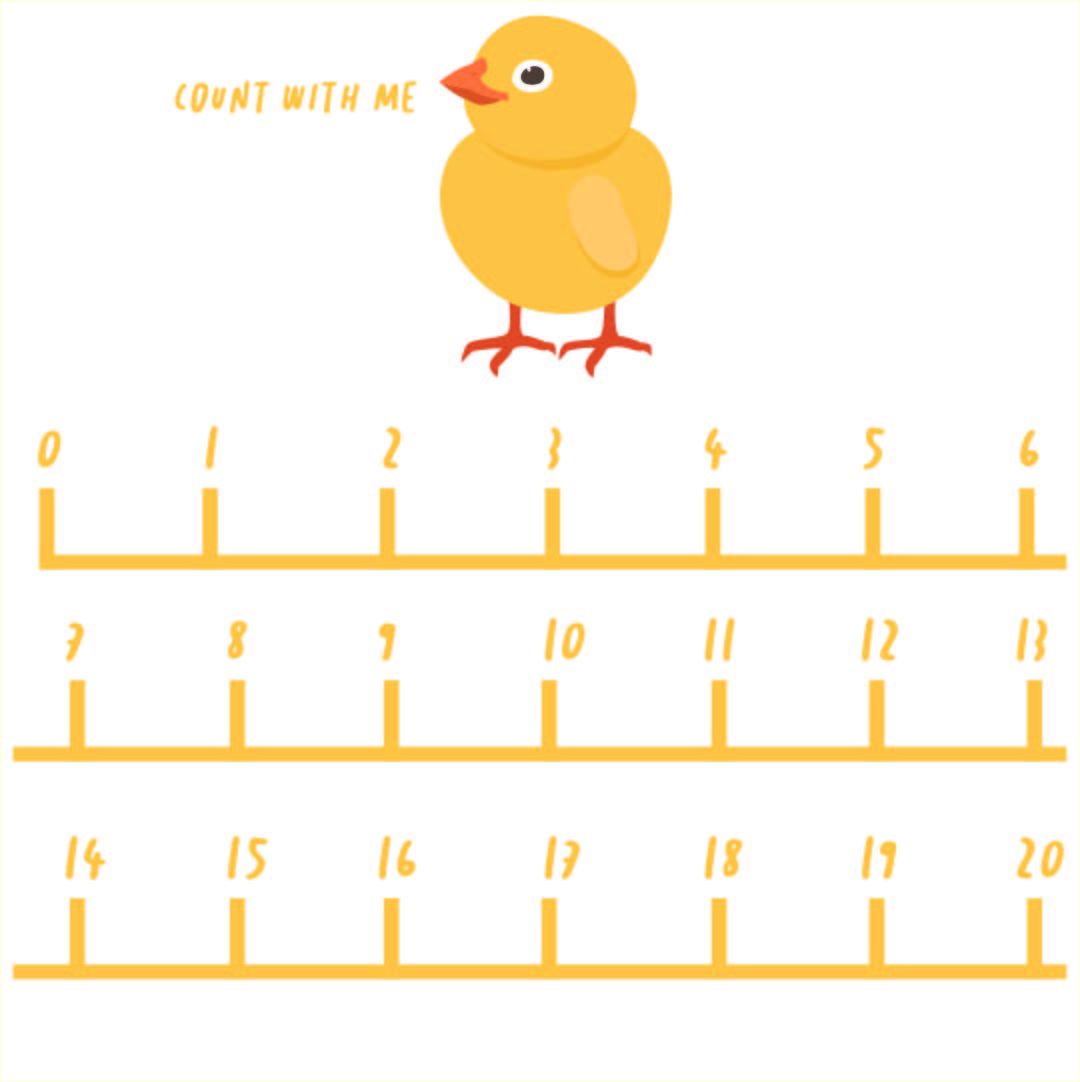### How do you make a number line?

A number line is a straight horizontal line in math where you place numbers at equal intervals along the length. It is useful to do simple math problems with small numbers, usually from 0 to 20 and you can also do it for negative numbers. However, if the number is bigger than 20 or a fraction, it will be harder to use this tool. Hence, when students first taught about it, they usually wouldn’t be asked to make a large number line. Here is a short step by step to make a basic number line.

1.    Draw a long line on your paper to make the base of the number line.
2.    Add hash marks on the line so it looks like a fence.
3.    Start writing the numbers below the hash marks. Start with the smallest number (for example 0) from the left and write the next number upon each hash mark.
4.    Stop writing at number 20 as it will be hard to do math problems with the method.

### What is the biggest number on the number line?

When students are first introduced to number lines, they usually will be shown a large number line that consists of 100 numbers. However, there is no biggest number in number lines because as you add more numbers to the right the number will always be bigger than before. For instance, 1.000.000 (1 million) is a big number but it cannot be the biggest number because 1 million+1 is bigger. Making a number line and writing numbers on it just make it easy to tell what number is bigger or smaller but it can never tell what the biggest number is.

### Does a number line go on forever?

A number line can go on forever and that’s why it has arrows on both sides. It can be a large number line that goes from -1.000.000 to 100 million and beyond. However, some people think that infinity is the end number of a number line. Whilst it is true there is no bigger number than infinity, but infinity itself is not a number hence it is not the end number of a number line.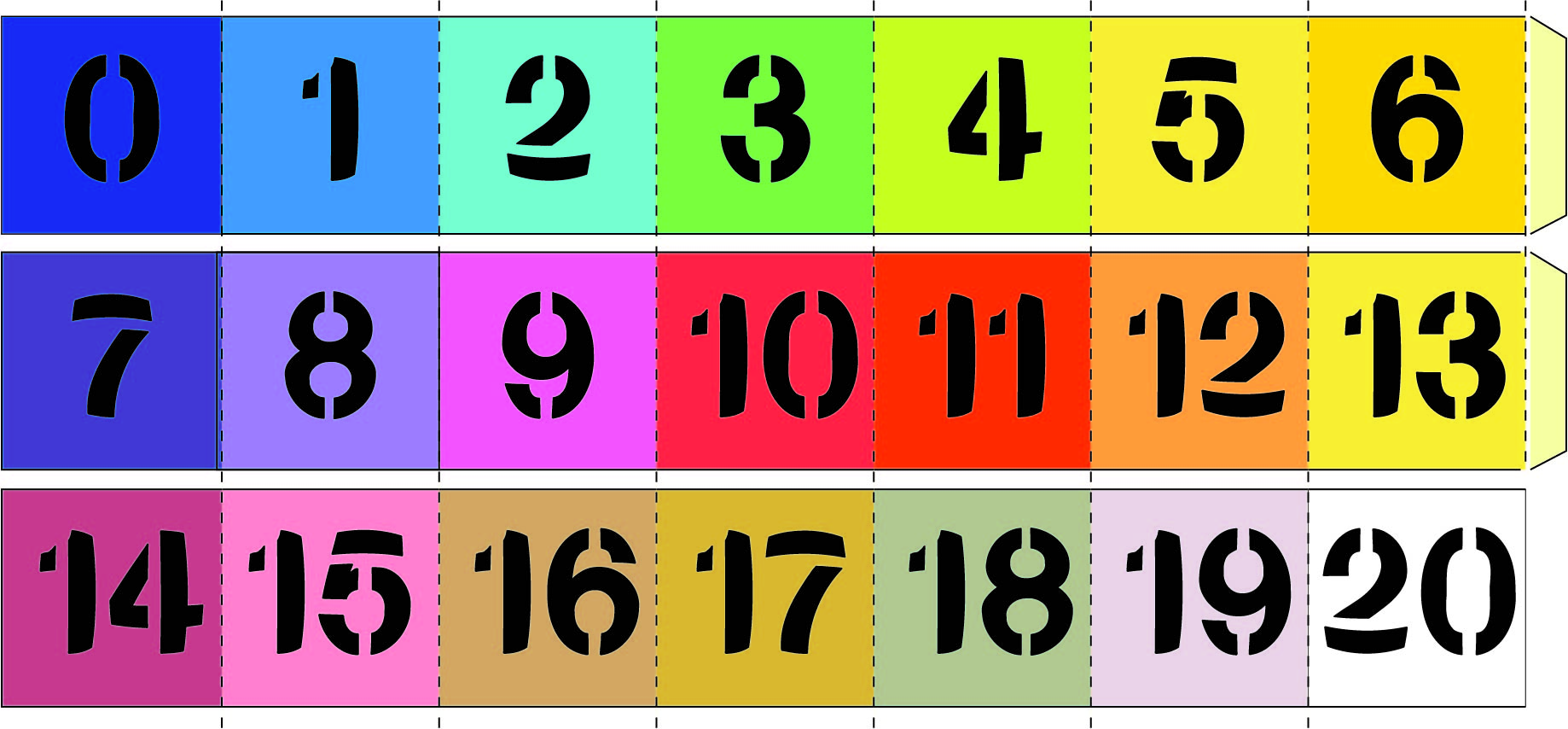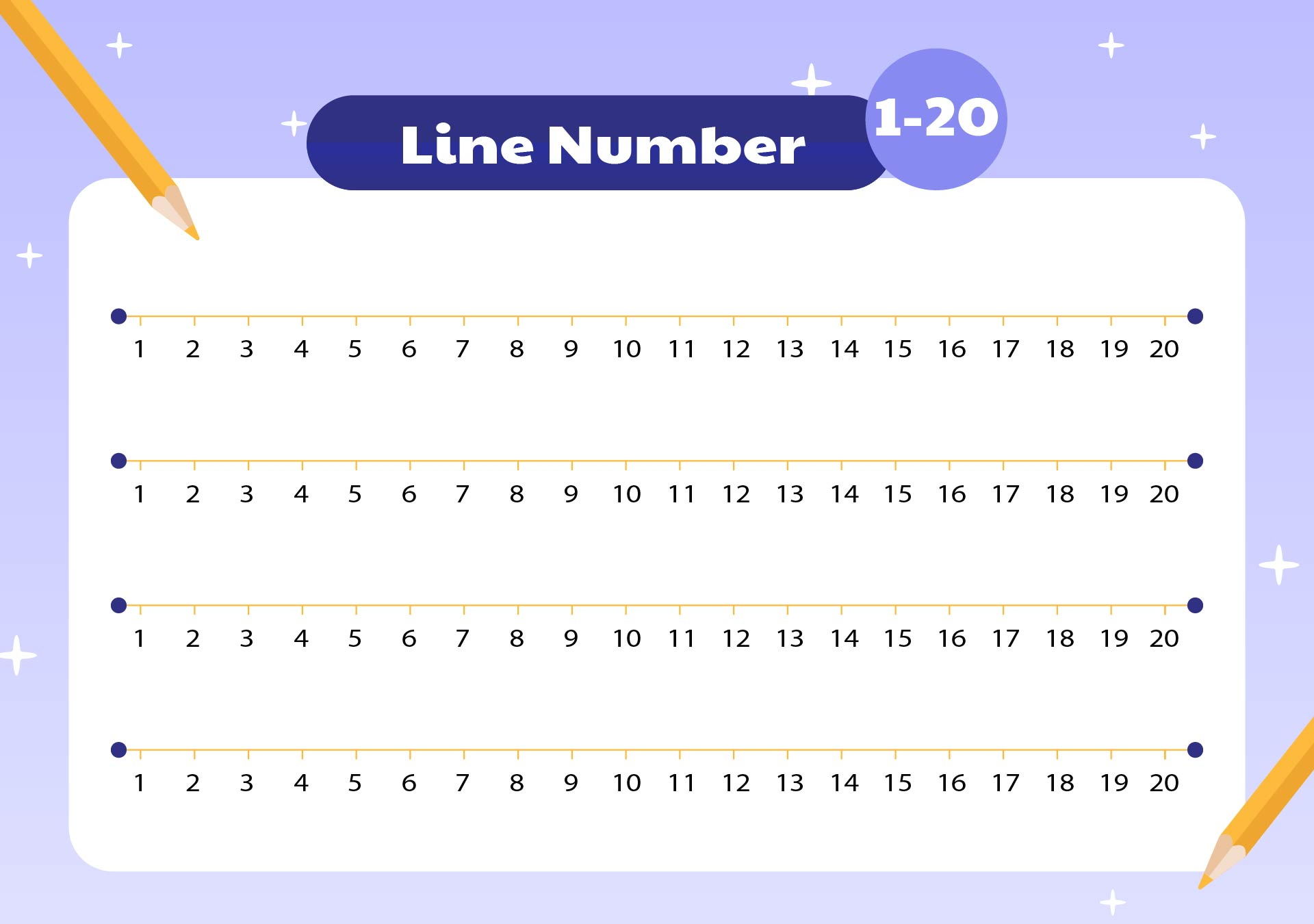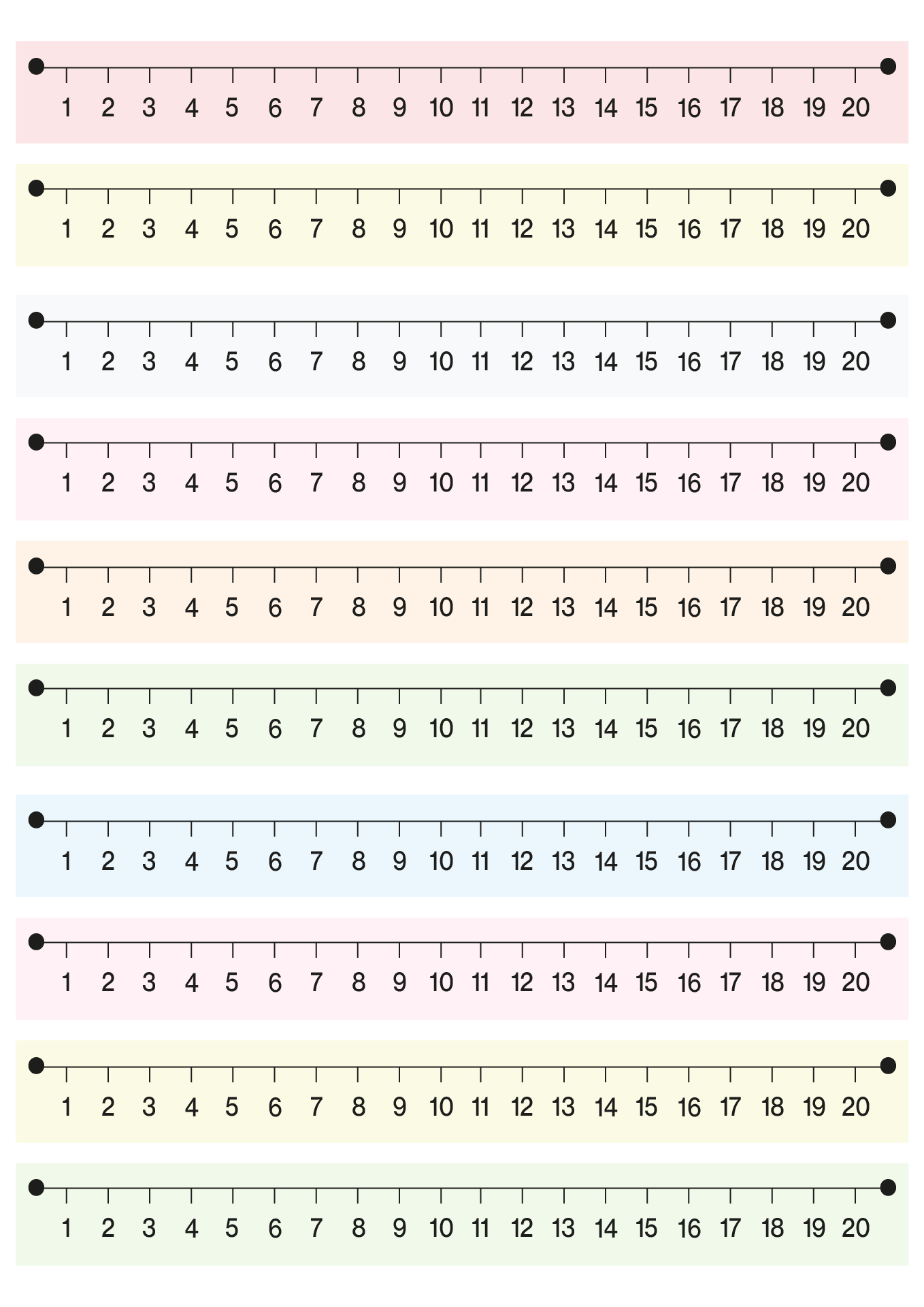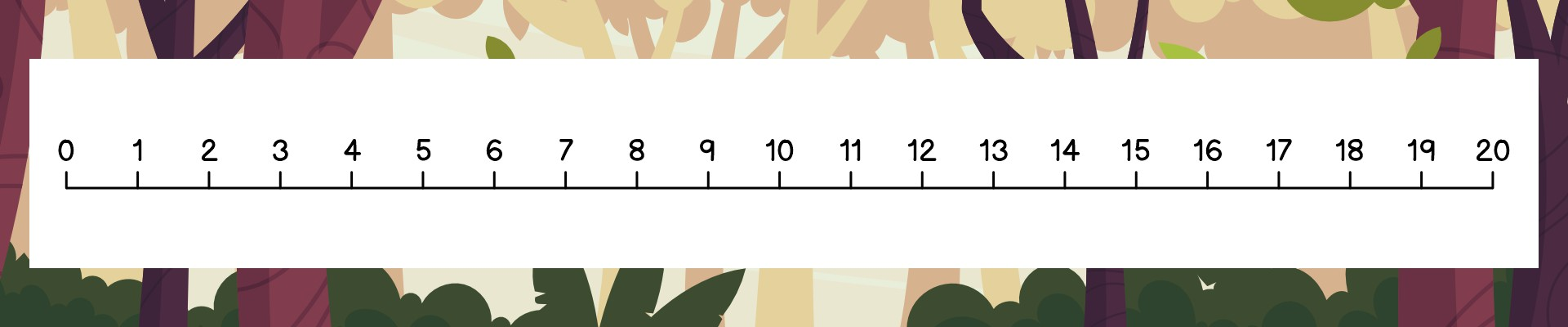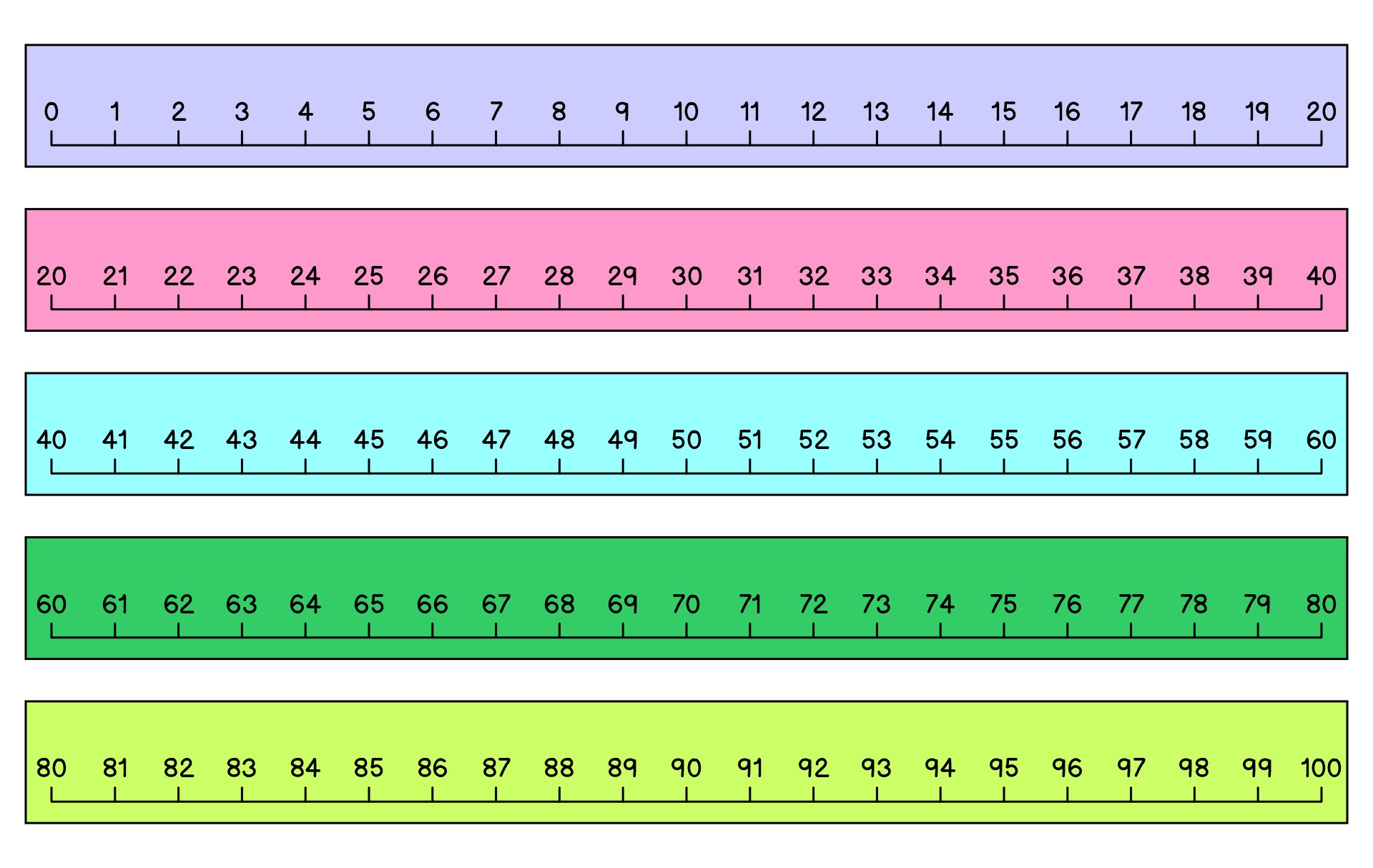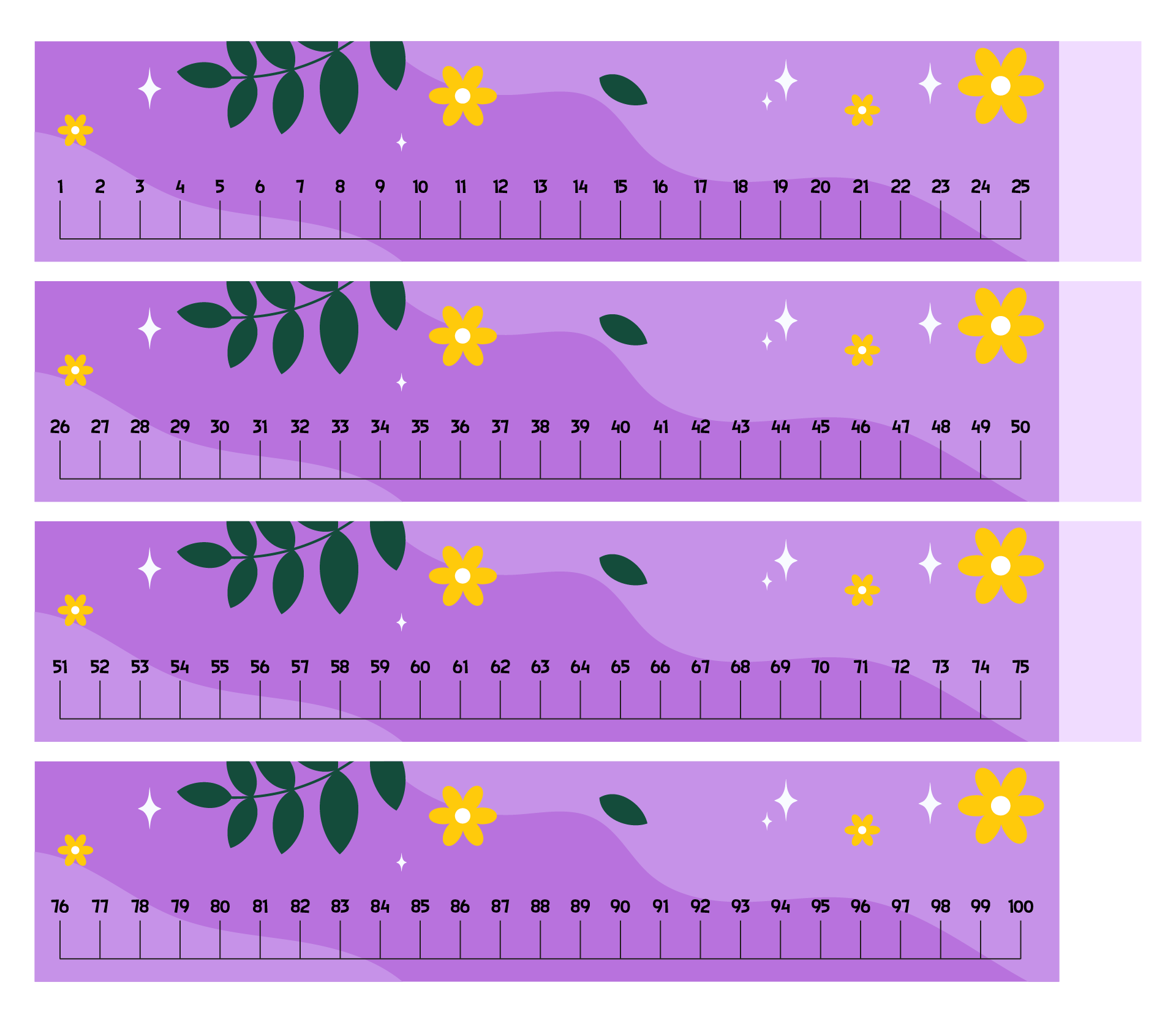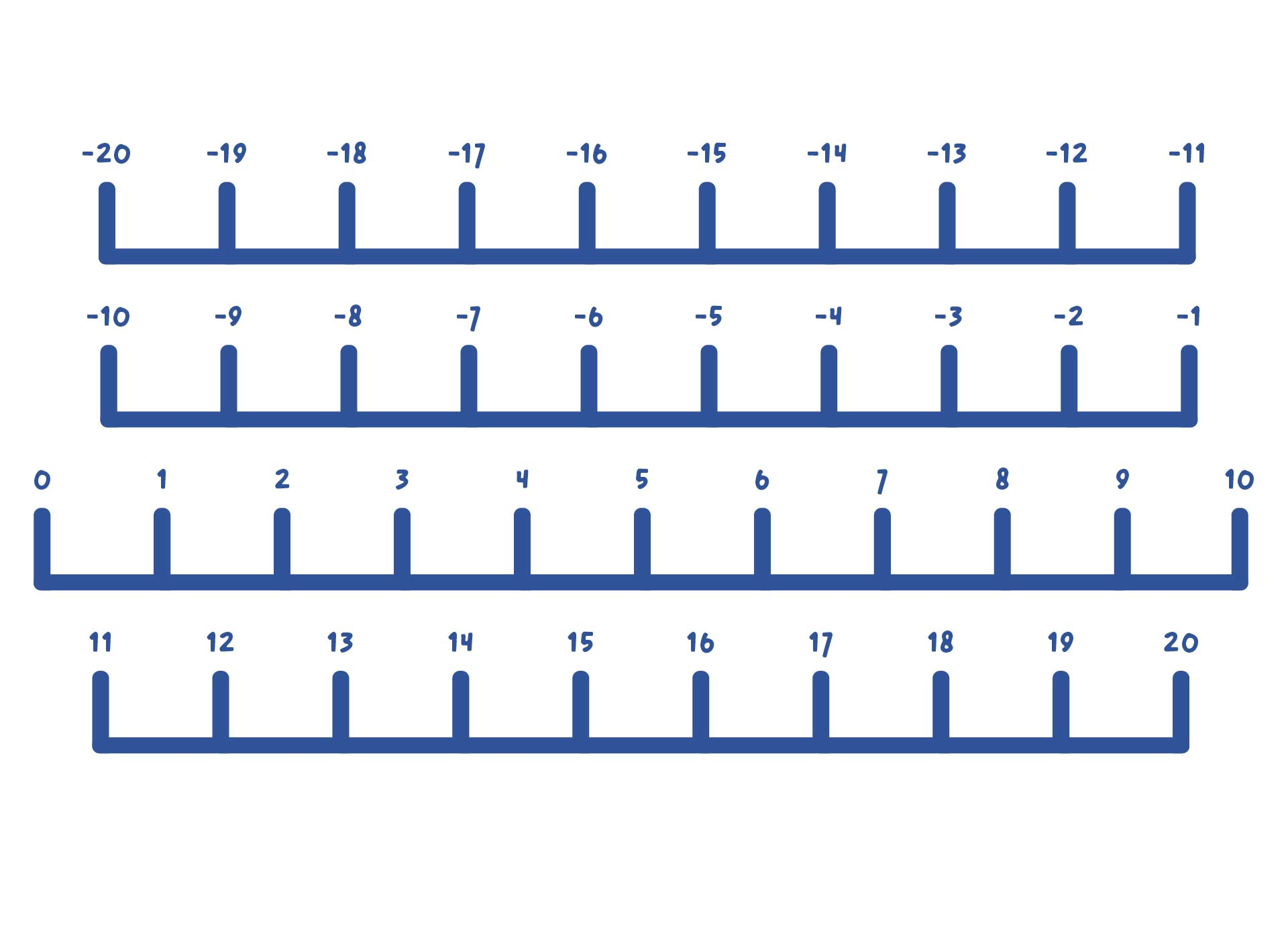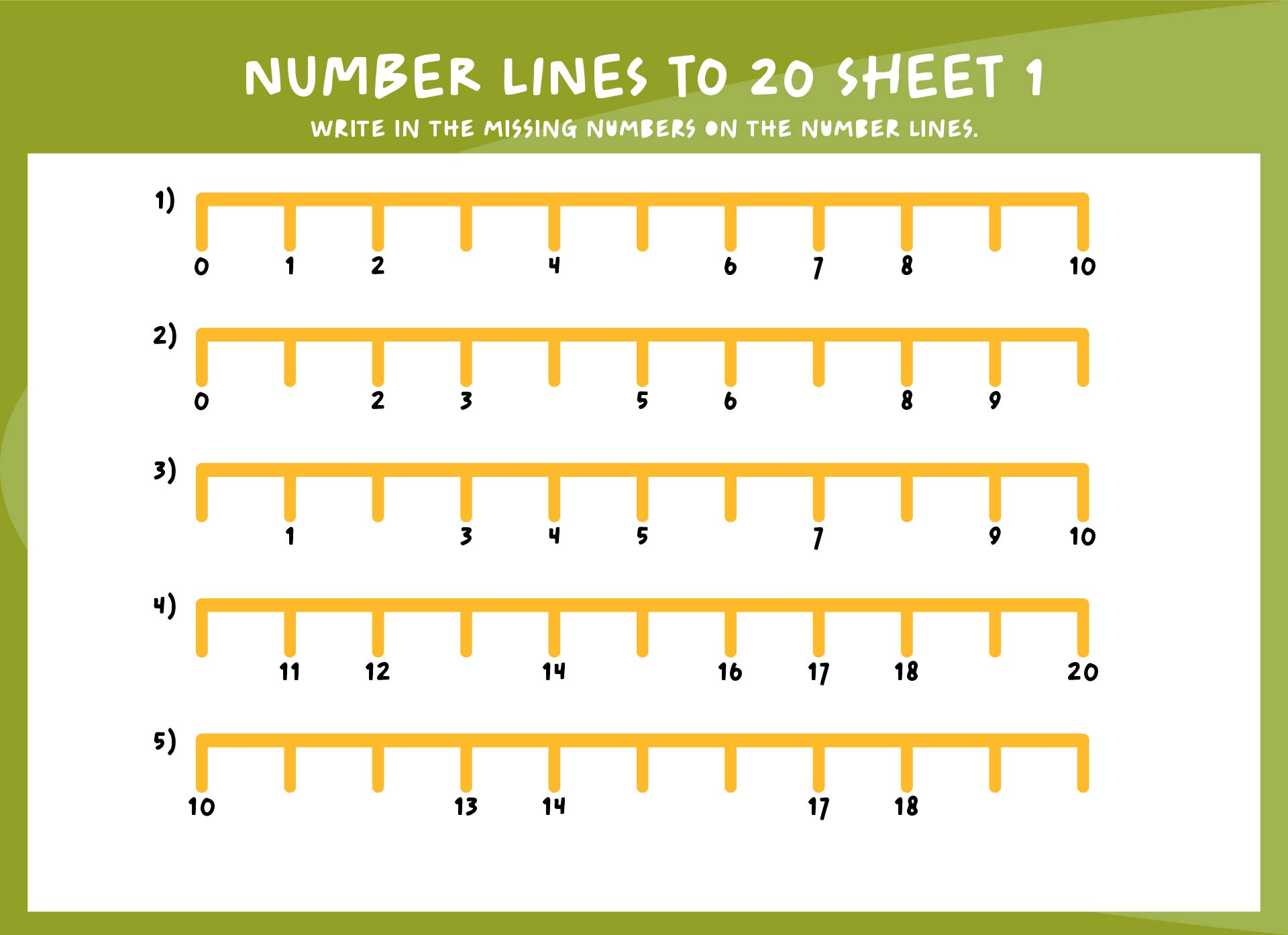### What is 3/4 on a number line?

The ¾ on a number line means fraction. When you draw a number line, there will be some spaces between the numbers but it doesn’t mean there is no number in those spaces. For example, the number between 0 and 1 is the fraction ½. The problem with fractions is, many students are confused when asked to place the fraction between numbers. For instance, when asked to put ½ on their number line they will put it between numbers 1 and 2 instead of 0 and 1. Thus, it is important to teach the number line in early children's education as they will use it later to learn Geometry and anything else in high school.